Home >> Class 9 >>

# Force and Laws of Motion Class 9 Science Chapter, Explanation, Notes, Question Answers

## CBSE Class 9 Science Chapter 9 Force and Laws of Motion, Explanation, Examples, Question Answers

Force and Laws of Motion CBSE Class 9 Science Chapter 9 - Complete explanation and Notes of the chapter 'Force and Laws of Motion'. Topics covered in the lesson are Introduction of Force, Newton’s third law of motion, Balanced and Unbalanced Forces, Law of conservation of momentum, Newton’s first law of motion, Newton’s second law of motion. Given here is the complete explanation of the chapter, along with all the important questions and NCERT solutions to book questions have also been provided for the ease of students.

### Class 9 Science Chapter 9 - Force and Laws of Motion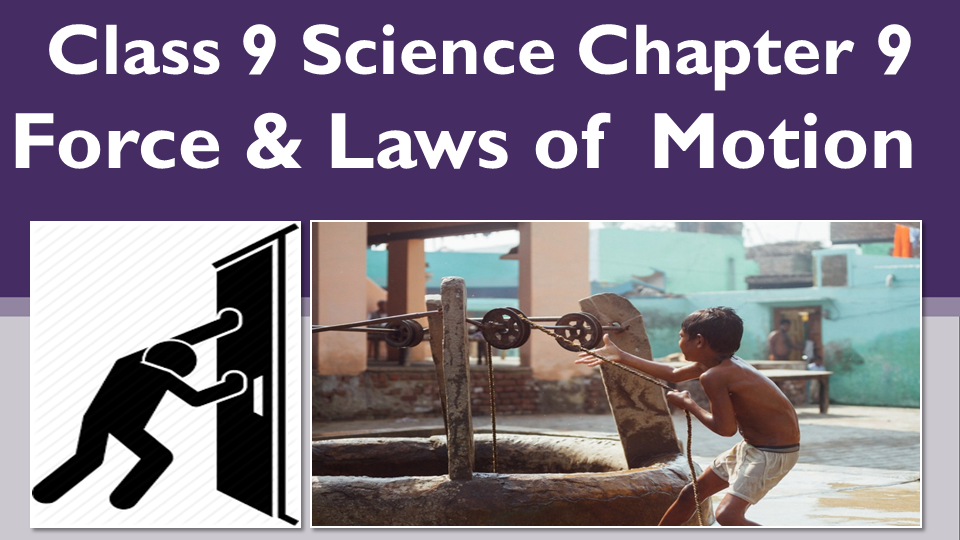## Introduction of force

As you all are familiar that if we want to open a door, we have to push the door handle. Similarly, when we want to close it, we have to pull it. This shows that in order to move the body we need to push or pull. This push or pull is called force. Likewise, if we need to hammer a nail in wood, we have to strike the hammer on nail with great strength.

So, combining both we can sum up that: The Pushing and Pulling or the Strength is force. We can define it in a technical language as   - An external influence which when applied on a body can bring about changes in it.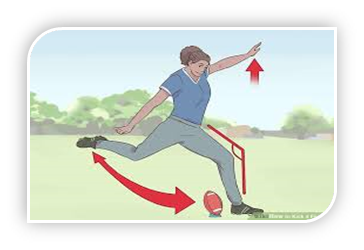The following changes can be brought about in a body:

Change in Position, Like: Kicking of Football

Change in Direction, Like: Applying Force on steering wheel of car

Change in Dimension, Squeezing a tube of toothpaste

Change in Speed of body, Accelerating a Car

### Types of forces - Balanced and unbalanced forces

We have two Types of Forces depending upon whether the effect of force is seen or felt or not . That is:

• Balanced force
• Unbalanced force

Balanced Force :  The equal and opposite force acting on a body, which will not make the body move.

For example: If we have a box, and in order to move it, we apply force. Let’s say, the same force is acting on an object from opposite sides. Then both forces will cancel each other’s effect as their magnitude is the same and both are acting from opposite sides. So, the resultant force will be zero.

Unbalanced Force: The unequal and opposite force acting on the body, which will make the body move in the direction of greater force applied.

For example: If we have a box, and in order to move it, we need to apply a force. Let’s say, the force of unequal magnitude is acting on an object from opposite sides. This time both the forces will not cancel each other effect as their magnitude is not the same. So, the resultant force will not be zero and will also make the body move in the direction of greater force applied.

Newton’s law of motion

There are certain laws given by Sir Isaac Newton which are obeyed by all bodies. Let’s start with Newton’s laws of motion now:

### Newton’s First Law of Motion

It states that a body at rest will remain at rest, a body in motion will remain in motion until or unless some external force is applied.

For Example: If we need to ride a bicycle we need to paddle as it can’t move on its own.  We have to apply force on paddles to ride it.

Similarly, if we need to change its direction we need to apply force on the steering wheel. The reason behind it is the inherent property called inertia.

Inertia

Let us try to understand it:  It is an inherent property that is present in all bodies from the time of their origin. That means this property is not fended into a substance, it is present from the time of its origin. This property actually resist any kind of change in body by itself like if we need to ride a bicycle, we have to paddle it. It will not move on its own. We need to apply force by paddling it.

Please note- In order to move or change any property of body, we need to overcome inertia.

There are certain factors on which inertia depends:

• It depends upon mass: More the mass, more is the inertia or lesser the mass, less is the inertia.

For example: We have two balls one heavier and one lighter. let’s say both the balls are rolling down a slope. In order to stop them we need to apply force. It is seen that to stop heavier ball we need to apply more force. The reason being that it is heavier, it has more Inertia. That means the resistance to change its state.

Types of Inertia

It is of three types:

• Inertia of Rest
• Inertia of Motion
• Inertia of direction

Inertia of Rest: Is the inability of body to change the state of rest on its own.

Inertia of Motion: Is the inability of body to resist the change in state of motion on its own.

Inertia of direction: Is the inability of body to resist the change in direction of body on its own.

Examples to understand more about Inertia

• Have you noticed that passengers in a bus fall forward when bus stops suddenly.

When the bus is moving, the bus and the passengers sitting in it are in motion. But when force is applied on the brakes, it stops, but due to inertia of motion the passengers remain in the state of motion, hence, fall forward.

• Have you experienced that when carpet is beaten with a stick, the dust separates out

Initially the carpet and dust are in state of rest. But when carpet is beaten with a stick, it comes in motion and moves backward. But due to inertia of rest, the  dust particles remain in the state of rest hence, get separated.

Have noticed that leaves and fruits falls down  when branch of tree is shaken vigorouslyInitially, the branch of a tree and its leaves and fruits are in state of rest. But when force is applied on the branch of a tree, the tree comes in motion but due to inertia of rest, the leaves and fruits remain in state of rest hence, falls down.

All the bodies in motion possess a physical property momentum .Let us learn about it before starting with Newton’s second law of motion.

Momentum: It is the measure of motion in body or It is equal to the product of mass and velocity of a body

Formula : p=m x v

Units = Kg m/s

It depends upon velocity. As we know, velocity is a vector quantity so as momentum is a vector quantity

• Have you noticed a karate player : He can break a pile of tiles with a single blow of his hand

The karate player strikes the pile of tiles with his hand very fast. In doing so the large momentum of his hand is reduced to zero in a very short duration.

### Newton’s second law of motion

Let’s learn about Newton’s Second Law of Motion now:

Newton’s Second Law of Motion: According to it, the rate of change of momentum of a body is directly proportional to the force applied.

Let us try to understand it mathematically

Consider a ball of mass “m” moving with initial velocity “u”. Then suddenly a retarding force “F” is applied and it is brought to rest, final velocity say “v”. Retardation “a” is produced in a ball.

Consider a body of mass = m

Travelling with initial velocity =u

Suddenly brakes are applied so, force exerted =F

Final velocity = v

Initial Momentum =mu

Final Momentum =mv

Change in Momentum=final momentum –initial momentum =v-u

Rate of change in Momentum=mv-mu/t

Then , according to second law it was said by Newton that:

Rate of change in Momentum is directly proportional to force applied that is :

Applying second law we get,

mv- mu/t ∝F

or, F ∝m(v-u)/t

As we know, from Newton’s first equation that v-u/t=a

So, substituting the value of v-u/t as “a” , we get :

or,F ∝m.a

Removing proportionality sign, we get :

F=K m .a (where K is a constant of proportionality and its value is taken as 1 .

If we talk about the S.I unit of Force, please write it down, it is kept after the name of Newton that is: S.I. unit of force is Newton.

We can define it as:

As we know F= m x a

So, putting units of all involved in the equation, we get :

F =m x a

1N=1Kg x 1m/sec2

It is defined as:

If we consider mass of body as 1kg and acceleration to be 1 meter per second square then we have : 1 Newton as: Force is said to be one Newton, when it acts on a body of mass 1kg, producing an acceleration of 1 meter per second square.

• Have you noticed that a cricket player lowers his hands while taking a catch

A fast moving ball has a large momentum. In stopping the cricket ball, the momentum of ball has to be reduced to zero. Now when the cricket player moves his hands back while taking a catch, he increases the time taken for rate of change of momentum. Therefore, less force is exerted on the hands of cricketer and hence, he doesn’t get hurt.

• Have you noticed that in athletics mostly high jumping athlete is provided either with cushion or a heap of sand on the ground to fall upon.

This is to prevent injury to the athlete when he falls down after a jump. When high jumper falls on soft landing site; the jumper takes a long time to come to a stop. So, the rate of change of momentum is less. Therefore, the force exerted by the ground on athlete is also less and he doesn’t get hurt.

• Have you noticed that when we push something harder, our hands also start paining.

Likewise, when we move on a road over a large distance, our feet also start paining.The reason is that: When we apply force on somebody, that body also exerts an equal and opposite force on us.

### Newton’s third law of motion

Let us learn about Newton’s Third Law of Motion now.

According to it  : For every action there is an equal and opposite reaction.

Suppose body A applies force on body B , then B also applies the force with the same magnitude but in opposite direction . So, mathematically we can write as : Fab=-Fba.

In this –ve sign shows the other force is in the opposite direction.

• Have you noticed that the gun also Recoils after firing a bullet

When a bullet is fired from a gun, it moves with certain velocity in forward direction. As a result, it applies equal & opposite force on gun.  Therefore, it recoils.

• Another important application is how we walk.

When we walk on the ground, our foot pushes the ground backward and in return, the ground pushes our foot forward. The forward reaction is exerted by the ground, on our foot which makes us walk forward. However, if the ground is slippery it becomes difficult to walk because on slippery ground, friction is less. Due to this, we can’t exert backward action which would produce a forward reaction.

### Law of Conservation of Momentum

As we have already discussed that all moving bodies possess momentum. So, it was seen that the momentum for a system is a constant quantity which is further explained by a law given below.

For a system, the sum total momentum is constant, provided no external force acts on the bodies. Or we can say that momentum is never created nor destroyed, it is always conserved.

Let’s try to explain it mathematically: For this we need to consider a system (means that any bodies that are considered for particular period). Like if we taken gun and bullet is fired from it then they both form the system. Similarly, if I say that a Car and a truck were moving on a road and both after certain period, collide, then both car and truck, form the system.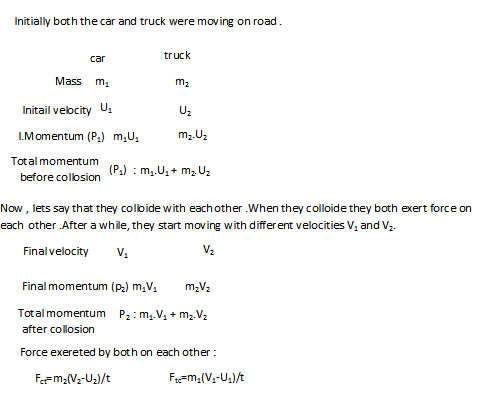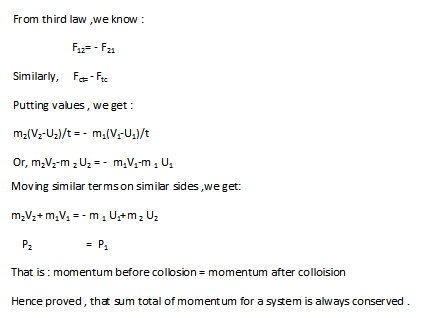• Have you noticed it is difficult for a fireman to hold a hose which ejects a large amount of water at high velocity.

Fireman finds it difficult to hold a hose pipe because as water moves through it with certain action, with a large velocity, in turn it produces large reaction.

• Have you seen a horse pulling a cart.

A horse pulls a cart by Action & Reaction because they are equal and opposite and are acting on different bodies & hence, do not cancel each other. Action is on ground and reaction is on horse and cart. Reaction is greater than friction & therefore, the horse & cart move forward due to an unbalanced force.

### NCERT Book Solutions

Q 1 . Which has more inertia?

• ?Rubber ball or stone of same size
• ?Bicycle? or train
• ?A 5 Rs coin or 1 Rs coin

Ans : (a) stone

(b) train

(c) Rs 5 coin

Q2: A football player kicks a football towards the goal .The goal keeper of the opposite team collects the football and kick it towards a player of his team. Also identify the agent supplying force in each case .

Ans : (i) Velocity changes from zero to maximum value when a football player kicks the ball towards the goal . Force is applied by the player in forward direction .

(ii) Velocity changes from maximum value to zero when the goalkeeper stops the football. In this case the force is applied by the goalkeeper in a direction opposite to the direction of motion of the football.

(iii) Velocity changes from zero to some maximum value when goalkeeper kicks it to a player of his team . The force in this case is applied again by a goalkeeper but in the direction directed from goalkeeper to player of his team .

Q3: Explain why some leaves detach from a tree when branch of tree is shaken ?

Ans : Initially, the branch of tree and its leaves and fruits are in state of rest. But when force is applied on the branch of a tree, the tree comes in motion but due to inertia of rest, the leaves and fruits remain in state of rest hence, falls down.

Q4: Why do you fall in forward direction when bus stops suddenly and also fall backwards when it starts suddenly?

Ans  : When bus is moving, the bus and the passengers sitting in it are in motion. But when force is applied on the brakes, it stops, but due to inertia of motion the passengers remain in the state of motion, hence, fall forward.

Q5: If action is always equal to reaction,explain how a horse can pull a cart ?

Ans : A horse pulls a cart by Action & Reaction because they are equal and opposite and are acting on different bodies & hence, do not cancel each other. Action is on ground and reaction is on horse and cart. Reaction is greater than friction & therefore, the horse & cart move forward due to an unbalanced force.

Q6: Explain why is it difficult for a fireman to hold a hose , which ejects large amounts of water at high velocity ?

Ans : Fireman finds it difficult to hold a hose pipe because as water moves through it with certain action, with a large velocity, in turn it produces large reaction.

Q7: From a rifle of mass 4kg , a bullet of mass 50g is fired with initial velocity of 35m/sec. Calculate the velocity with which gun recoils ?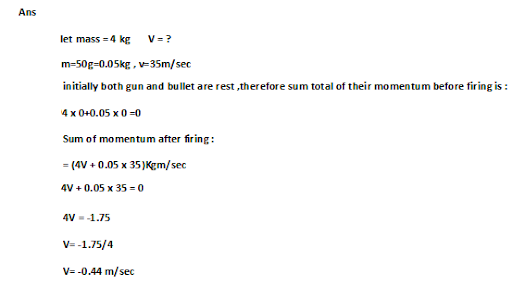Q8: Two objects of 100g and 200g are moving along the same line and their velocities are 2m/sec and 1 m/sec. They collide and after collision first objects move with velocity 1.67m/sec. Determine the velocity of the second object?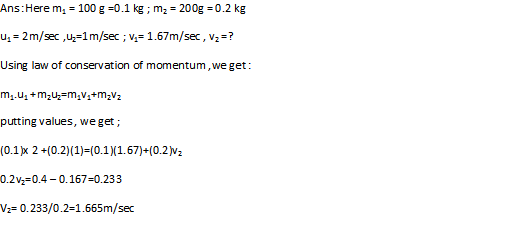Q9: An object experiences a net zero external unbalanced force .Is it possible for the object to be travelling with a non zero velocity ?If yes, state the conditions that must be placed on the magnitude and direction of velocity .If no, provide a reason ?

Ans: Yes , it can move with the uniform velocity in accordance with Newton’s first law of motion .

Q10: When a carpet is beaten with sticks, dust comes out. Explain?

Ans : Initially the carpet and dust are in state of rest. But when carpet is beaten with a stick, it comes in motion and moves backward. But due to inertia of rest, the dust particles remain in the state of rest hence, get separated.

Q11: Why is it advised to tie luggage kept on a roof of a bus with a rope ?

Ans : If the luggage is not tied with a rope ,the luggage will move forward due to inertia or motion when the brakes are applied to the bus . The luggage will move backwards when the bus suddenly starts moving .The luggage will move forward and backward when bus changes the velocity and the luggage may fall down from the bus.

Q12: A batsman pushes a cricket ball which then roll on a level ground .After covering a short distance ,the ball comes to rest .The ball slows to a stop because :

1. The batsman did not hit the ball hard enough .
1. Velocity is proportional to the force exerted on the ball.
1. There is a force on the ball opposing the motion .
1. There is an unbalanced force on the ball,so the ball would want to come to rest .

Ans: (c)  There is a force on the ball opposing the motion .

Q13: A truck starts from rest and rolls down a hill with constant acceleration .It travels a distance of 400 m in 20 sec .Find its acceleration .Find the force acting on it if its mass is 7 metric tonnes?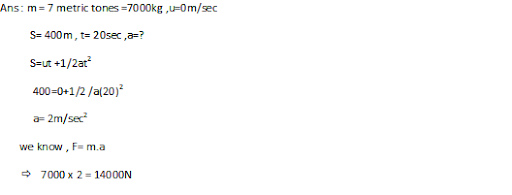Q14: A stone of 1 kg is thrown with velocity of 20m/sec across the frozen surface of a lake and comes to rest after travelling a distance of 50m .What is the force of friction between the stone and an ice ?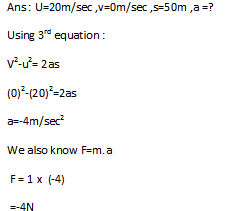Q15: A 8000kg engine pulls a train of 5 wagons, each of 2000kg, along a horizontal track. If the engine exerts a force of 40,000N and track offers a frictional force of 5000N, Calculate

1. Accelerating force
1. Acceleration of train
1. Force of wagon 1 on 2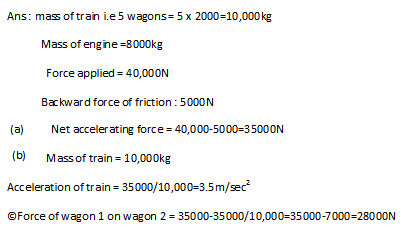Q16: An automobile vehicle has a mass of 1500 kg , what must be the force between vehicle and the road if the vehicle is to be stopped with negative acceleration of 1.7m/sec2?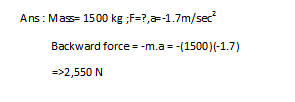Q17: What is the momentum of an object of mass m, moving with velocity v ?

1. (mv)2(b) mv2(c)1/2mv2 (d) mv

Ans : (d) m v

Q18: Using a horizontal  force of 200N, we intend to move a wooden cabinet across a floor at a constant velocity .what is the frictional force that will be exerted on the cabinet ?

Ans : when the cabinet is to be moved with constant velocity that is with 0 acceleration ,the force applied must just overcome friction .Force of friction =force applied =200N

Q19: Two objects each of mass of 1.5kg are moving in the same straight line but in the opposite direction. The velocity of each object is 2.5m/sec before the collision during which they stick together .What will be the velocity of combined object after the collision?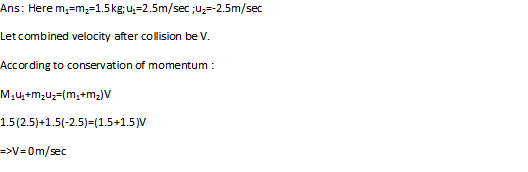Q20: A hockey ball of mass 200g travelling at 10m/sec is struck by hockey stick so as to return it along the original path with the velocity of 5m/sec. calculate the change in momentum that occurred in motion of hockey ball by the force applied by hockey stick?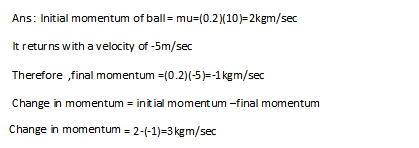Q21: A bullet of mass 10g travelling horizontally with a velocity of 150m/sec strikes a stationary wooden block and comes to rest in 0.03s.Calculate the distance of penetration of the bullet into the block .Also calculate the magnitude of the force exerted by the wooden block on the bullet ?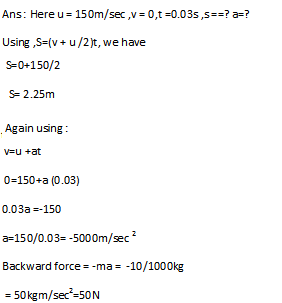Q22: An object of mass 1kg travelling in a straight line with velocity of 10m/sec collides and sticks to a stationary wooden block of mass 5 kg .Calculate the total momentum, just before the impact and just after an impact. Also, find the velocity of combined object?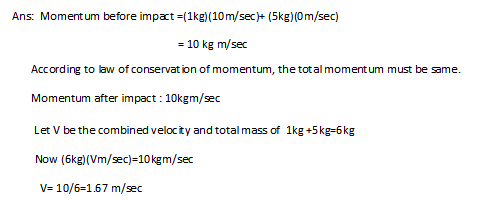Q23: An object of mass 100kg is accelerated uniformly from a velocity of 5m/sec to 8m/sec in 6 sec .Calculate the initial and final momentum of the object. Also, find the magnitude  of force exerted on the object ?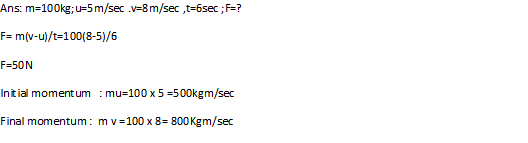Q24: How much momentum will a dumb bell of mass 10kg transfer to the floor if it falls from a height of 80cm ?Take its downward acceleration o be 10m/sec 2?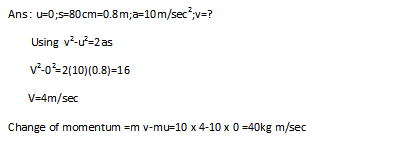Q25: A hammer of mass 500 g, moving at 50 m/sec, strikes a nail. The nail stops the hammer in a very short time of 0.01sec. What is the force of nail on the hammer?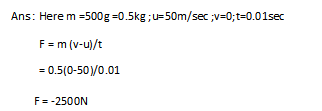Q26: A motor car of mass 1200kg is moving along a straight line with a uniform velocity of 90km/hr. Its velocity is slowed down to 18km/hr by unbalanced force. Calculate the acceleration and change in momentum. Also ,calculate the force required ?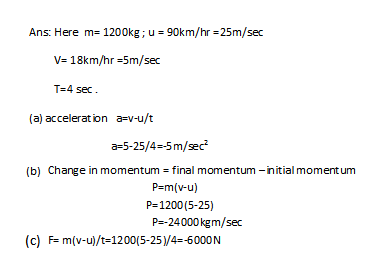Q27: A large truck and a car both moving with the same velocity v, have a headon collision and both of them come to halt after that.  If the collision lasts for 1 sec :

1. Which vehicle experiences greater force or impact?
2. Which vehicle experiences greater change in momentum?
3. Which vehicle experiences greater acceleration?
4. Why is the car likely to suffer more damage than the truck?

Ans :

1. Both will experience equal force of impact in accordance with Newton’s third law .
2. Both will experience equal change in momentum
3. Car will experience greater acceleration because mass of car is less
4. Car will suffer more damage due to greater retardation.